# Euclid's Division Lemma

Euclid's Division Lemma
Go back to  'Integers'

In this mini-lesson, we will learn about the Euclid division lemma by understanding the Euclid division algorithm, the division using Euclid division lemma, and how to apply them while solving problems. We will also discover interesting facts around them.

Paul wants to plant some saplings in his backyard.

He has 48 sapling plants with him.He is making 9 rows such that he can plant 5 saplings in each row.

If he plants the saplings in the above way, he can plant a total of $$5 \times 9 = 45$$ sapling plants.

The sapling plants remaining with him would be $$48 - 45 = 3$$

We can write the above scenario mathematically as: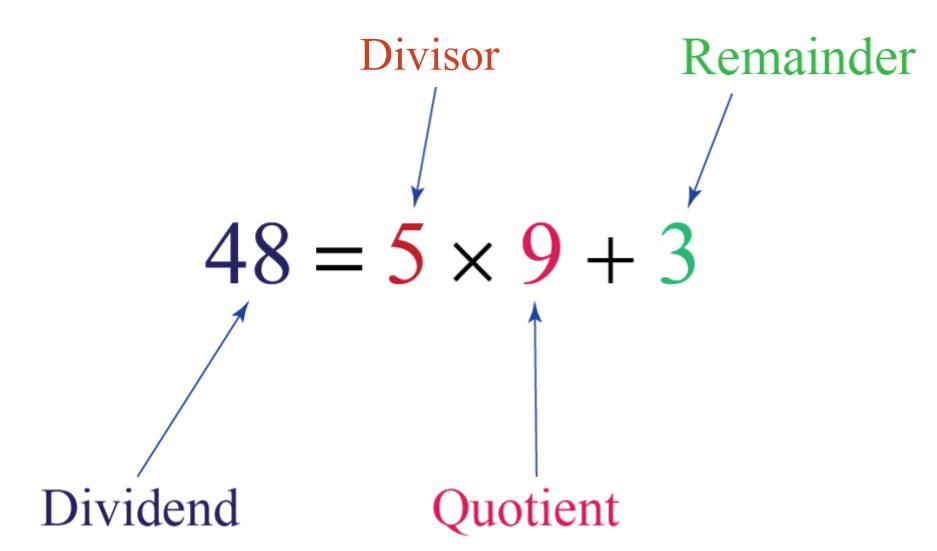The above scenario shows the way of representing the division of positive integers with the help of Euclid's division lemma.

Now let's explore Euclid's division lemma in this lesson.

## Lesson Plan

 1 What Is Euclid’s Division Lemma? 2 Thinking Out of the Box! 3 Solved Examples on Euclid's Division Lemma 4 Important Notes on Euclid's Division Lemma 5 Interactive Questions on Euclid's Division Lemma

## What Is Euclid’s Division Lemma?

Euclid’s Division Lemma (lemma is similar to a theorem) says that given two positive integers, $$a$$ and $$b$$, there exists unique integers, $$q$$ and $$r$$, such that:

$\boxed{a = bq + r,\;0 \leqslant r < b}$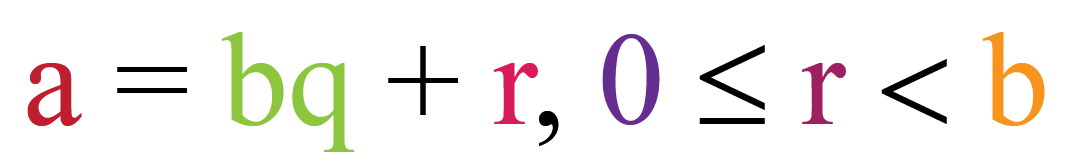The integer $$q$$ is the quotient and the integer $$r$$ is the remainder.

The quotient and the remainder are unique.

In simple words, Euclid's Division Lemma statement is that if we divide an integer by another integer (non-zero integer), we will get a unique integer as qoutient and a unique integer as remainder

Here are some examples of the application of this lemma:

$23 = 2 \times 11 + 1 \\[0.2cm] 54 = 7 \times 7 + 5 \\[0.2cm] 63 = 9 \times 7 + 0$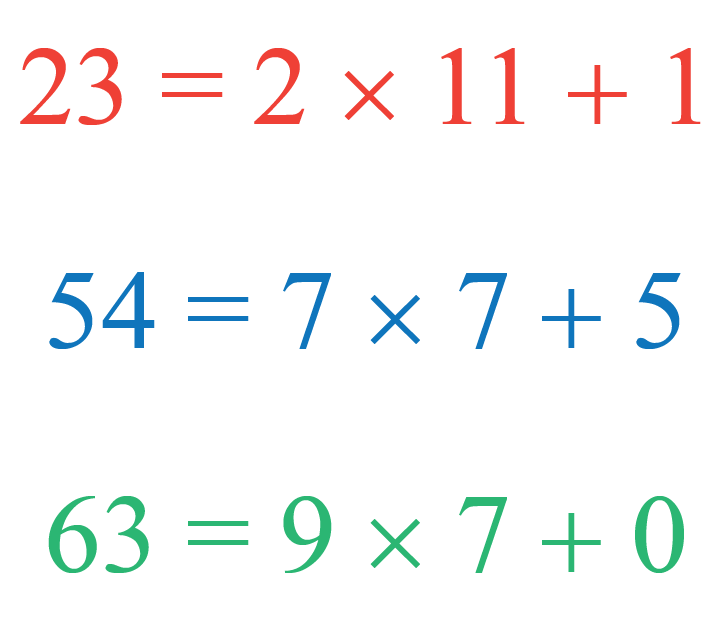## How to Divide Integers Using Euclid’s Lemma?

Euclid’s lemma or Euclid's Division Lemma statement says that for given two positive integers, $$a$$ and $$b$$, there exists unique integers $$q$$ and $$r$$ such that:

$a = bq + r,\;0 \leqslant r < b$

So, let's take $$a = 9$$ and $$b = 1$$

$9 = 1 \times 9 + 0$

Here, $$q = 9$$ and $$r = 0$$, we can clearly see that $$0 \leqslant r < 1$$

Now, let's take $$a = 9$$ and $$b = 2$$

$9 = 2 \times 4 + 1$

Here, $$q = 4$$ and $$r = 1$$, we can clearly see that $$0 \leqslant r < 2$$

Now, let's take $$a = 9$$ and $$b = 3$$

$9 = 3 \times 3 + 0$

Here, $$q = 3$$ and $$r = 3$$, we can clearly see that $$0 \leqslant r < 3$$

Now, let's take $$a = 9$$ and $$b = 4$$

$9 = 4 \times 2 + 1$

Here, $$q = 2$$ and $$r = 1$$, we can clearly see that $$0 \leqslant r < 4$$

We can observe that when we divide two integers using the Euclid lemma division algorithm, we can always get a non-negative integral remainder which is always less than the divisor.

## What Is the Use of Euclid's Division Lemma?

Euclid's division lemma has many uses.

Let's learn about two of the most important uses of this algorithm.

### Calculating HCF of Two Numbers

We use Euclid's division lemma to find the HCF of large numbers which is typically difficult to calculate using basic HCF calculation techniques.

The HCF of two numbers can be calculated with the help of Euclid's division lemma by following these steps.

Let's take two numbers for which we need to find the HCF.

$$c$$ and $$d$$ are two numbers such that $$c > d$$.

Step 1:- Apply Euclid’s division lemma to $$c$$ and $$d$$. We can find whole numbers, $$q$$ and $$r$$ such that $$c = dq + r,\;0 \leqslant r < d$$.

Step 2:- If $$r = 0$$, $$d$$ is the HCF of $$c$$ and $$d$$. If $$r \neq 0$$, apply the division lemma to $$d$$ and $$r$$.

Step 3:- Continue the process till the remainder is zero. The divisor at this stage will be the required HCF.

### Example

Use Euclid’s algorithm to find the HCF of 4052 and 12576.

### Solution

We can observe that 12576 > 4052, hence $$c = 12576$$ and $$d = 4052$$.

According to Euclid's division lemma, we have:

$12576 = 4052 \times 3 + 420$

Here the remainder $$420 \neq 0$$, we again apply Euclid's division lemma to 4052 and 420

$4052 = 420 \times 9 + 272$

Let's repeat the same process until we get the remainder 0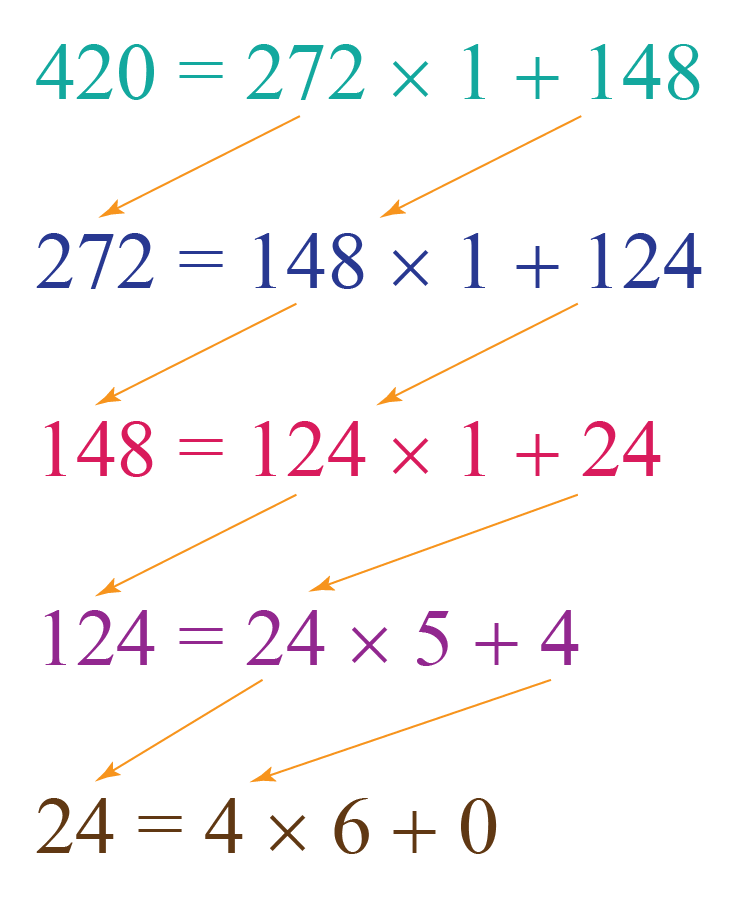Since the remainder is now zero, we can stop the process.

The divisor at this stage is 4; the HCF of 12576 and 4052 is 4

Explore the HCF calculator below to find the HCF of any two numbers using Euclid's division lemma.

Finding Properties of Numbers

We can understand the various properties of odd and even numbers using Euclid's division lemma.

### Example

Show that every positive even integer is of the form $$2q$$ and that every positive odd integer is of the form $$2q + 1$$, where $$q$$ is some integer.

Solution

Assume $$a$$ is a positive integer and $$b = 2$$.

By Euclid’s algorithm, we can write:

$a = 2q + r$

Where $$q \geq 0$$ and $$r$$ can take either $$0$$ or $$1$$ by the rule $$0 \leqslant r < b$$

Thus, $$a = 2q$$ or $$a = 2q + 1$$.

If $$a$$ is of the form $$2q$$, then $$a$$ is an even integer, and if $$a$$ is of the form $$2q + 1$$, then it is an odd integer.

For example, if we substitute $$q = 1, 2$$, and $$3$$ then $$a = 2q$$ is even

$$a = 2 \times 1 = 2$$
$$a = 2 \times 2 = 4$$
$$a = 2 \times 3 = 6$$

And, if we substitute $$q = 1, 2$$, and $$3$$ then $$a = 2q + 1$$ is odd

$$a = 2 \times 1 + 1= 3$$
$$a = 2 \times 2 + 1= 5$$
$$a = 2 \times 3 + 1= 7$$Think Tank
1. Does Euclid’s Division Lemma hold if $$a$$ is a negative integer?
2. Find any positive even integer of the form $$6q$$, $$6q + 2$$ or $$6q + 4$$ where $$q$$ is some integer.

## Solved Examples on Euclid's Division Lemma

 Example 1

Paul knows that $$n$$ is an odd integer.

He wants to prove that $$n^2 - 1$$ is a multiple of 8

Can you help him?

Solution

By Euclid’s Division Lemma, $$n$$ can be written as:

$n = 2k + 1,\;k \in \mathbb{Z}$

Thus,

\begin{align}&{n^2} - 1 \;= {\left( {2k + 1} \right)^2} - 1\\& \qquad\quad= \left( {4{k^2} + 4k + 1} \right) - 1\\& \qquad\quad= 4{k^2} + 4k\\&\qquad\quad = 4k\left( {k + 1} \right) & \end{align}

Note that the product $$k(k+1)$$ will always be even.

This is because either $$k$$ is even, or $$k + 1$$ is even.

Thus,

$$4k(k + 1)$$, must be a multiple of 8.

 $\therefore n^2 - 1 \text{ is a multiple of 8 }$
 Example 2

Paul is emptying two water tanks that are filled with water using a jug.

The water tanks have capacities of 420 liters and 130 liters.

What is the maximum capacity of the jug he should use so that no water remains in the tanks?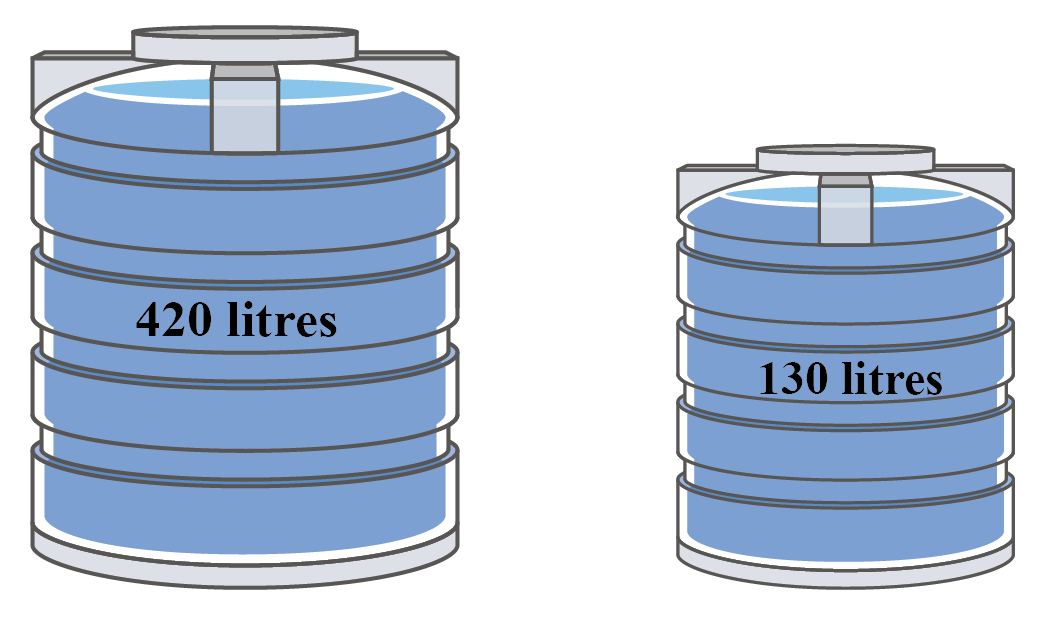Solution

Given:

The capacities of tanks are 420 liters and 130 liters.

To find the maximum capacity of the jug, we need to find the HCF of 420 and 130.

Let's apply Euclid's division lemma to calculate the HCF of these numbers.

$420 = 130 \times 3 + 30 \\[0.2cm] 130 = 30 \times 4 + 10 \\[0.2cm] 30 = 10 \times 3 + 0$

The HCF of 420 and 130 is 10.

 $$\therefore$$ Maximum capacity of the jug is 10 liters.
 Example 3

The cube of a positive integer is divided by 9.

What can be the possible remainders?

Solution

We have to use Euclid's Division Lemma to find the remainders.

If we can express the cube of any positive integer in the form $$9q + r$$, then we can solve the problem easily.

We will then have to find what values $$r$$ can take.

Let $$n$$ be any positive integer.

Note that when we divide $$n$$ by 3, we can get three possible remainders: 0, 1, or 2.

Thus, $$n$$ can be written as:

\begin{align}&n = 3k\\&\quad{\rm{or}}\\&n = 3k + 1\\&\quad{\rm{or}}\\&n = 3k + 2\end{align}

Let's consider each case separately.

Case 1: In this case, we have:

${n^3} = {\left( {3k} \right)^3} = 27{k^3} = 9\left( {3{k^3}} \right)$

Thus, the cube of $$n$$ will be perfectly divisible by 9 in this case (the remainder will be 0).

Case 2: In this case, we have:

\begin{align}&{n^3} = {\left( {3k + 1} \right)^3}\\&\quad = {\left( {3k} \right)^3} + {\left( 1 \right)^3} + 3\left( {3k} \right)\left( 1 \right)\left( {3k + 1} \right)\\&\quad= 27{k^3} + 1 + 9k\left( {3k + 1} \right)\\&\quad = 9k\left( {3{k^2} + 3k + 1} \right) + 1\\&\quad = 9\left\{ {k\left( {3{k^2} + 3k + 1} \right)} \right\} + 1 & \end{align}

It is clear that on dividing the cube of $$n$$ by 9, in this case, we will be left with a remainder of 1.

Case 3: In this case, we have:

\begin{align}&{n^3} = {\left( {3k + 2} \right)^3}\\&\quad= {\left( {3k} \right)^3} + {\left( 2 \right)^3} + 3\left( {3k} \right)\left( 2 \right)\left( {3k + 2} \right)\\&\quad = 27{k^3} + 8 + 18k\left( {k + 2} \right)\\&\quad= 9k\left( {3{k^2} + 2k + 4} \right) + 8\\&\quad = 9\left\{ {k\left( {3{k^2} + 2k + 4} \right)} \right\} + 8 & \end{align}

In this case, on dividing the cube of $$n$$ by 9, we will be left with a remainder of 8.

Thus, we conclude that dividing the cube of a positive integer by 9 can yield three possible remainders:
$\boxed{{\text{Three Possible Remainders: }}0,1,8}$

In other words, the cube of any integer can be expressed as follows:

\begin{align}&{n^3} = 9k\\&\quad\;{\rm{or}}\\&{n^3} = 9k + 1\\&\quad\;{\rm{or}}\\&{n^3} = 9k + 8\end{align}

You can verify this result by taking some cubes and checking the remainders you obtain upon their division by 9.

 $\therefore \text{Possible remainders are 0, 1, 8}$
Download Arithmetic Integers Worksheets
Arithmetic Integers
grade 10 | Questions Set 2
Arithmetic Integers
grade 10 | Answers Set 2
Arithmetic Integers
grade 10 | Questions Set 1
Arithmetic Integers
grade 10 | Answers Set 1

More Important Topics
Numbers
Algebra
Geometry
Measurement
Money
Data
Trigonometry
Calculus